## Global and Local Coordinate Systems

### Global Coordinate System

The global coordinate system describes the arena in which your radar or sonar simulation takes place. Within this arena, you can place radar or sonar transmitters and receivers, and targets. These objects can be either stationary or moving. You specify the location and motion of these objects in global coordinates.

You can model the motion of all objects using the `phased.Platform` System object™. This System object computes the position and speed of objects using constant-velocity or constant-acceleration models.

You can model the signals that propagate between objects in your scenario. The ray paths that connect transmitters, targets, and receivers are specified in global coordinates. You can propagate signals using these System objects: `phased.FreeSpace`, `phased.WidebandFreeSpace`, `phased.LOSChannel`, or `phased.WidebandLOSChannel`. If you model two-ray multipath propagation using `twoRayChannel` (Radar Toolbox), the boundary plane is set at z = 0 in the global coordinate system.

### Local Coordinate Systems

When signals interact with sensors or targets, the interaction is almost always specified as a function of the sensor or target local coordinates. Local coordinate systems are fixed to the antennas and microphones, phased arrays, and targets. They move and rotate with the object. Local coordinates are commonly adapted to the shape and symmetry of the object.

Because signals propagate in the global coordinate system, you need to be able to convert local coordinates to the global coordinates. You do this by constructing a 3-by-3 orthonormal matrix of coordinate axes. The matrix columns represent the three orthogonal direction vectors of the local coordinates expressed in the global coordinate system. The coordinate axes of a local coordinate system must be orthonormal, but they need not be parallel to the global coordinate axes.

When you need to compute the range and arrival angles of a signal, you can use the `rangeangle` function. When you call this function with the source and receiver position expressed in global coordinates, the function returns the range and arrival angles, azimuth and elevation, with respect to the axes of the global system. However, when you pass the orientation matrix as an additional argument, the azimuth and elevation are now defined with respect to the local coordinate system.

You use local coordinates to specify

• the location and orientation of antenna or microphone elements of an array. The beam pattern of an antenna array depends upon the angle of arrival or emission of radiation with respect to the array local coordinates.

• the reflected energy from a target is a function of the incident and reflection angles with respect to the target local coordinate axes.

Two examples of local coordinate systems are

• an airplane may have a local coordinate system with the x-axis aligned along the fuselage axis of the body and the y-axis pointing along the port wing. Choose the z-axis to form a right-handed coordinate system.

• a vehicle-mounted planar phased array may have a local coordinate system adapted to the array. The x-axis of the coordinate system may point along the array normal vector.

The following figure illustrates the relationship of local and global coordinate systems in a bistatic radar scenario. The thick solid lines represent the coordinate axes of the global coordinate system. There are two phased arrays: a 5-by-5 transmitting uniform rectangular array (URA) and 5-by-5 receiving URA. Each of the phased arrays carries its own local coordinate system. The target, indicated by the red arrow, also carries a local coordinate system.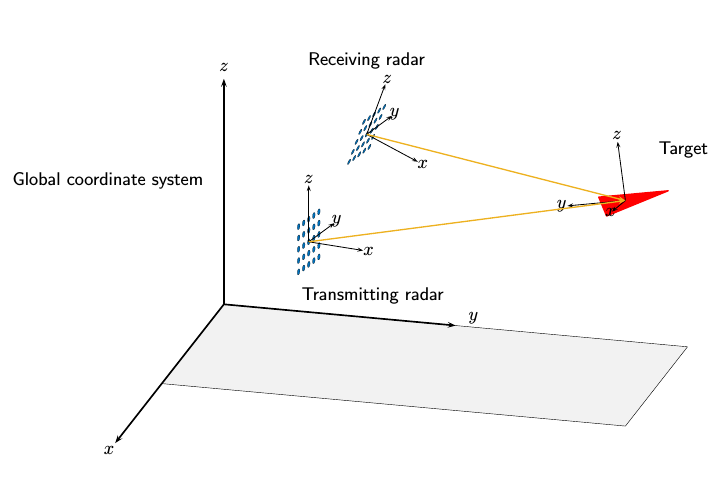The next few sections review the local coordinate systems used by arrays.

#### Local Coordinate Systems of Arrays

The positions of the elements of any Phased Array System Toolbox™ array are always defined in a local coordinate system. When you use any of the System objects that create uniform arrays, the array element positions are defined automatically with respect to a predefined local coordinate system. The arrays for which this property holds are the `phased.ULA`, `phased.URA`, `phased.UCA`, `phased.HeterogeneousULA`, and `phased.HeterogeneousURA` System objects. For these System objects, the arrays are described using a few parameters such as element spacing and number of elements. The positions of the elements are then defined with respect to the array origin located at (0,0,0) which is the geometric center of the array. The geometric center is a good approximation to the array phase center. The phase center of an array is the point from which the radiating waves appear to emanate when observed in the far field. For example, for a ULA with an odd number of elements, the elements are located at distances (-2d,-d,0,d,2d) along the array axis.

There are array System objects for which you must explicitly specify the element coordinates. You can use these objects for creating arbitrary array shapes. These objects are the `phased.ConformalArray` and `phased.HeterogeneousConformalArray` System objects. For these arrays, the phase center of the array need not coincide with the array origin or geometric center.

Element Boresight Directions.  In addition to element positions, you need to specify the element orientations, that is, the directions in which the elements point. Some elements are highly directional — most of their radiated energy flows in one direction, called the main response axis (MRA). Others are omnidirectional. Element orientation is the pointing direction of the MRA. You specify element orientation using azimuth and elevation in the array local coordinate system. The direction that an antenna or microphone MRA faces when transmitting or receiving a signal is also called the boresight or look direction. For the uniform arrays, all boresight directions of all elements are determined by array parameters. For conformal arrays, you specify the boresight direction of each element independently.

#### Local Coordinate System of Uniform Linear Array

Array Origin and Phase Center.  A uniform linear array (ULA) is an array of antenna or microphone elements that are equidistantly spaced along a straight line. In the Phased Array System Toolbox, the `phased.ULA` System object creates a ULA array. The geometry of the ULA orientation of its elements is determined by three parameters: the number of elements, the distance between elements, and the `ArrayAxis` property. For the ULA, the local coordinate system is adapted to the array — the elements are automatically assigned positions in the local coordinate system.

The positions of elements in the array are determined by the `ArrayAxis` property which can take the values `'x'`, `'y'` or `'z'`. The array axis property determines the axis on which all elements are defined. For example, when the `ArrayAxis` property is set to `'x'`, the array elements lie along the x-axis. The elements are positioned symmetrically with respect to the origin. Therefore, the geometric center of the array lies at the origin of the coordinate system.

This figure shows a four-element ULA with directional elements in a local right-handed coordinate system. The elements lie on the y-axis with their boresight axes pointing in the x-direction. In this case, the `ArrayAxis` property is set to `'y`.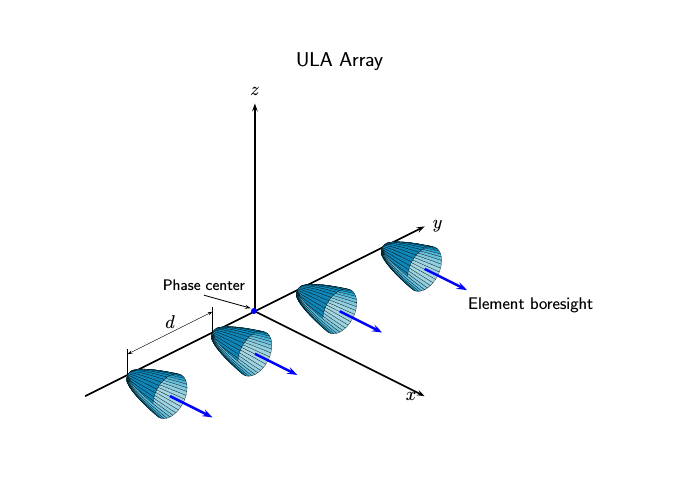ULA Element Boresight Direction.  In a ULA, the boresight directions of every element point in the same direction. The direction is orthogonal to the array axis. This direction depends upon the choice of `ArrayAxis` property.

ArrayAxis Property ValueElement Positions and Boresight Directions
`'x'`Array elements lie on the x-axis. Element boresight vectors point along the y-axis.
`'y'`Array elements lie on the y-axis. Element boresight vectors point along the x-axis.
`'z'`Array elements lie on the z-axis. Element boresight vectors point along the x-axis.

Local Coordinates Adapted to Uniform Linear Array

Construct two examples of a uniform linear array and display the coordinates of the elements with respect to the local coordinate systems defined by the arrays.

First, construct a 4-element ULA with one-half meter element spacing.

```sULA = phased.ULA('NumElements',4,'ElementSpacing',0.5); ElementLocs = getElementPosition(sULA)```
```ElementLocs = 3×4 0 0 0 0 -0.7500 -0.2500 0.2500 0.7500 0 0 0 0 ```
`viewArray(sULA)`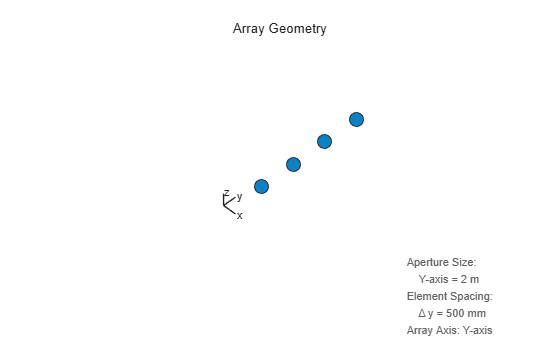The origin of the array-centric local coordinate system is set to the phase center of the array. The phase center is the average value of the array element positions.

`disp(mean(ElementLocs'))`
``` 0 0 0 ```

Because the array has an even number of elements, no element of the array actually lies at the phase center (0,0,0).

Next construct a 5-element ULA with thirty-centimeter element spacing.

```sULA1 = phased.ULA('NumElements',5,'ElementSpacing',0.3); ElementLocs = getElementPosition(sULA1)```
```ElementLocs = 3×5 0 0 0 0 0 -0.6000 -0.3000 0 0.3000 0.6000 0 0 0 0 0 ```
`viewArray(sULA1)`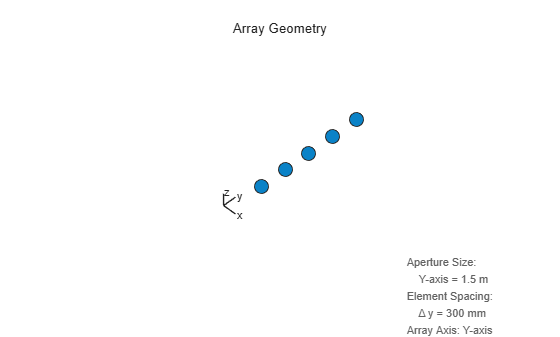Because the array has an odd number of elements in each row and column, the center element of the array lies at the phase center.

#### Local Coordinate System of Uniform Rectangular Array

Array Origin and Phase Center.  A uniform rectangular array (URA) is an array of antenna or microphone elements placed on a regular two-dimensional grid. The geometry of a URA and the location and orientation of its elements are determined by several parameters: the dimensions of the array, the distance between elements, and the `ArrayNormal` property. For the URA, the local coordinate system is adapted to the array — the elements are automatically assigned positions in the local coordinate system. The origin of the local coordinate system is the geometric center of the array. The phase center of the array coincides with the geometric center. The elements are automatically assigned positions in this local coordinate system. The positions are determined by the `ArrayNormal` property which can take the values `'x'`, `'y'` or `'z'`. All elements lie in a plane passing through the origin and orthogonal to the axis specified in this property. For example, when the `ArrayNormal` property is set to `'x'`, the array elements lie in the yz-plane as shown in the figure. The figure shows a 2-by-4 element URA with elements spaced d meters apart in both the y and z directions.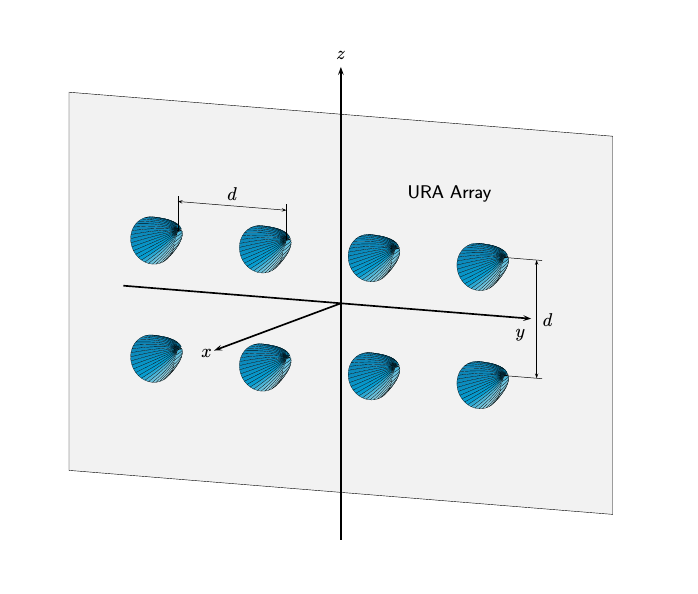Element Boresight Direction.  In a URA, like the ULA, the boresight directions of every element point in the same direction. You control this direction using the `ArrayNormal` property. For the URA shown in the preceding figure, the `ArrayNormal` property is set to `'x'`. Then, element boresights point along the x-axis.

ArrayNormal Property ValueElement Positions and Boresight Directions
`'x'`Array elements lie on the yz-plane. Element boresight vectors point along the x-axis.
`'y'`Array elements lie on the zx-plane. Element boresight vectors point along the y-axis.
`'z'`Array elements lie on the xy-plane. Element boresight vectors point along the z-axis.

Local Coordinates Adapted to Uniform Rectangular Array

Construct two examples of uniform rectangular arrays and display the coordinates of the elements with respect to the local coordinate systems defined by the arrays.

First, construct a 2-by-4 URA with one-half meter element spacing.

```sURA = phased.URA('Size',[2 4],'ElementSpacing',[0.5 0.5]); ElementLocs = getElementPosition(sURA)```
```ElementLocs = 3×8 0 0 0 0 0 0 0 0 -0.7500 -0.7500 -0.2500 -0.2500 0.2500 0.2500 0.7500 0.7500 0.2500 -0.2500 0.2500 -0.2500 0.2500 -0.2500 0.2500 -0.2500 ```
`viewArray(sURA)`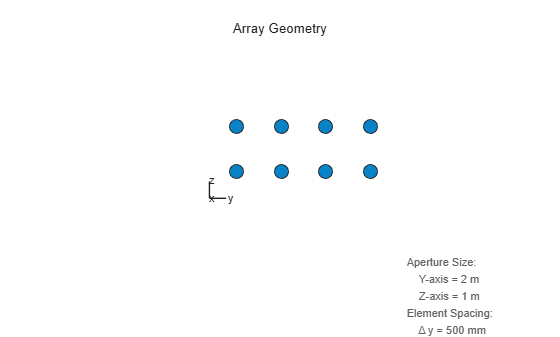The phase center of the array is the mean value of the array element positions. The origin of the array local coordinate system is set to the phase center of the array.

`disp(mean(ElementLocs'))`
``` 0 0 0 ```

Because the array has an even number of elements in each row and column, no element of the array actually lies at the phase center (0,0,0).

Next construct a 5-by-3 URA with thirty-centimeter element spacing.

```sURA1 = phased.URA([5 3],'ElementSpacing',[0.3 0.3]); ElementLocs = getElementPosition(sURA1)```
```ElementLocs = 3×15 0 0 0 0 0 0 0 0 0 0 0 0 0 0 0 -0.3000 -0.3000 -0.3000 -0.3000 -0.3000 0 0 0 0 0 0.3000 0.3000 0.3000 0.3000 0.3000 0.6000 0.3000 0 -0.3000 -0.6000 0.6000 0.3000 0 -0.3000 -0.6000 0.6000 0.3000 0 -0.3000 -0.6000 ```
`viewArray(sURA1)`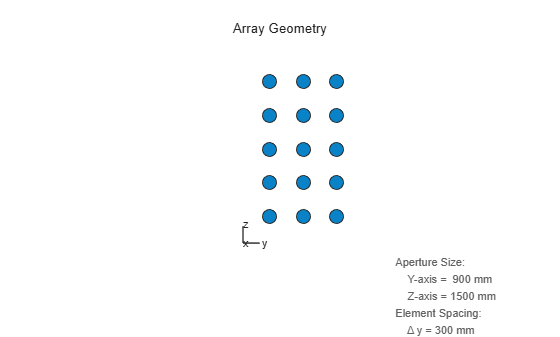Because the array has an odd number of elements in each row and column, the center element of the array lies at the phase center.

A signal arrives at the array from a point 1000 meters from along the +x-axis of the global coordinate system. The local URA array is rotated 30 degrees clockwise around the y-axis. Compute the angle of arrival of the signal in the local array axes.

```laxes = roty(30); [rng,ang] = rangeangle([1000,0,0]',[0,0,0]',laxes)```
```rng = 1.0000e+03 ```
```ang = 2×1 0 30.0000 ```

#### Local Coordinate System of Uniform Circular Array

Array Origin and Phase Center.  A uniform circular array (UCA) is an array of antenna or microphone elements spaced at equal angles around a circle. The `phased.UCA` System object creates a special case of a UCA. In this case, element boresight directions point away from the array origin like spokes of a wheel. The origin of the local coordinate system is the geometric center of the array. The geometry of the UCA and the location and orientation of its elements is determined by three parameters: the radius of the array, the number of elements, and the `ArrayNormal` property. The elements are automatically assigned positions in the local coordinate system. The positions are determined by the `ArrayNormal` property which can take the values `'x'`, `'y'` or `'z'`. All elements lie in a plane passing through the origin and orthogonal to the axis specified in this property. The phase center of the array coincides with the geometric center. For example, when the `ArrayNormal` property is set to `'x'`, the array elements lie in the yz-plane as shown in the figure. You can create a more general UCA with arbitrary boresight directions using the `phased.ConformalArray` System object.

This figure shows an 8-element UCA with elements lying in the yz plane.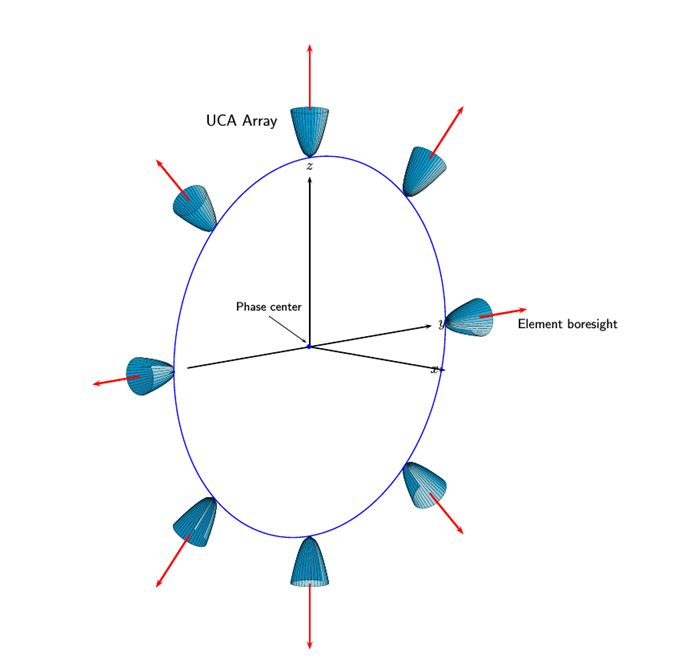Element Boresight Directions.  In a UCA defined by a `phased.UCA` System object, element boresight directions point radially outward from the array origin. In the UCA shown in the preceding figure, because the `ArrayNormal` property is set to `'x'`, the element boresight directions point radially outward in the yz-plane.

ArrayNormal Property ValueElement Positions and Boresight Directions
`'x'`Array elements lie on the yz-plane. All element boresight vectors lie in the yz-plane and point radially-outward from the origin.
`'y'`Array elements lie on the zx-plane. All element boresight vectors lie in the zx-plane and point radially-outward from the origin.
`'z'`Array elements lie on the xy-plane. All element boresight vectors lie in the xy-plane and point radially-outward from the origin.

#### Local Coordinate System of Conformal Arrays

Array Origin and Phase Center.  You can use `phased.ConformalArray` to create arrays of arbitrary shape. Unlike the case of uniform arrays, you must specify the element positions explicitly. An N-element array requires the specification of N 3-D coordinates in the array local coordinate system. The origin of a conformal array can be located at any arbitrary point. The boresight directions of the elements of a conformal array need not be parallel. The azimuth and elevation angles defining the boresight directions are with respect to the local coordinate system. The phase center of the array does not need to coincide with the geometric center. The same properties apply to the `phased.HeterogeneousConformalArray` array.

This illustration shows the positions and orientations of a 4-element conformal array.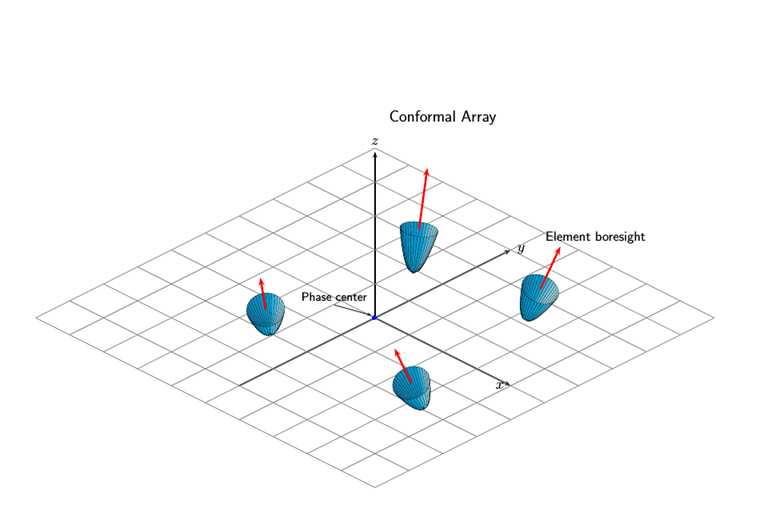4-Element Conformal Array

Construct a 4-element array using the ConformalArray System object™. Assume the operating frequency is 900 MHz. Display the array geometry and normal vectors.

```fc = 900e6; c = physconst('LightSpeed'); lam = c/fc; x = [1.0,-.5,0,.8]*lam/2; y = [-.4,-1,.5,1.5]*lam/2; z = [-.3,.3,0.4,0]*lam/2; sIso = phased.CosineAntennaElement(... 'FrequencyRange',[0,1e9]); nv = [-140,-140,90,90;80,80,80,80]; sConformArray = phased.ConformalArray('Element',sIso,... 'ElementPosition',[x;y;z],... 'ElementNormal',nv); pos = getElementPosition(sConformArray)```
```pos = 3×4 0.1666 -0.0833 0 0.1332 -0.0666 -0.1666 0.0833 0.2498 -0.0500 0.0500 0.0666 0 ```
`normvec = getElementNormal(sConformArray)`
```normvec = 2×4 -140 -140 90 90 80 80 80 80 ```
`viewArray(sConformArray,'ShowIndex','All','ShowNormal',true)`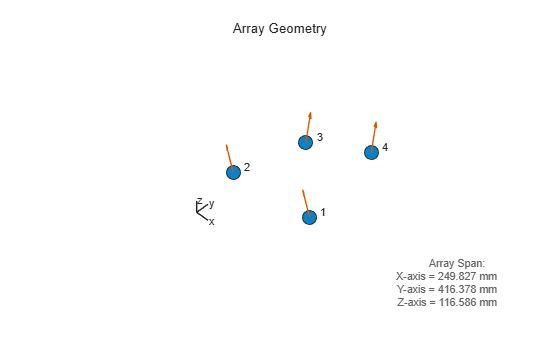### Converting Between Global and Local Coordinate Systems

In many array processing applications, it is necessary to convert between global and local coordinates. Two utility functions, `global2localcoord` and `local2globalcoord`, perform these conversions.

### Convert Local Spherical Coordinates to Global Rectangular Coordinates

Determine the position of a target in rectangular coordinates in the global coordinate system. First, specify the local spherical coordinates of a target with respect to a URA. The center of the URA defines the origin of the local coordinate system. The target location in local spherical coordinates is 30° azimuth, 45° elevation, and 1000 m range. To convert to global rectangular coordinates, specify the position of the local coordinate system origin in global coordinates. The origin of the local coordinate system is (1000,500,100) m from the global origin.

Convert the coordinates of the point to global rectangular coordinates. To convert from local spherical coordinates to global rectangular coordinates, use the `'sr'` option in the call to the `local2globalcoord` function.

`gCoord = local2globalcoord([30; 45; 1000],'sr',[1000; 500; 100])`
```gCoord = 3×1 103 × 1.6124 0.8536 0.8071 ```

The target is located at (1612,854,807) m in the global coordinate system.

### Convert Global Rectangular Coordinates to Local Spherical Coordinates

Determine the position of a target in local spherical coordinates centered at the phase center of a URA array. The center of the URA defines the origin of the local coordinate system and has the global rectangular coordinates (5000,3000,50). The local coordinate axes of the URA are (0,1,0), (1,0,0), and (0,0,-1). Specify the global rectangular coordinates of the target at (1000,500,10).

Convert the coordinates of the target to local spherical rectangular coordinates. To convert from global rectangular coordinates to local spherical coordinates, use the `'rs'` option in the call to the `global2localcoord` function.

```lCoord = global2localcoord([5000; 3000; 50],'rs',[1000; 500; 100], ... [0 1 0; 1 0 0; 0 0 -1])```
```lCoord = 3×1 103 × 0.0580 0.0006 4.7173 ```

The output has the form (az,el,rng). The target is located in local spherical coordinates at 58° azimuth, 0.6° elevation and 4717 m.